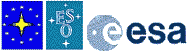How Does GPS Work?

The triangulation method

Now that you know the main principles of GPS, you may wish to learn more about the fundamental physics and mathematics behind GPS. Although it is not possible to go into great detail - the entire system is quite complex and many different aspects must be taken into account - here are some of the underlying facts.

The basic principle inherent in GPS is to determine with the best possible accuracy a point in space, as defined by three coordinates, here geographical latitude and longitude, as well as elevation above sea level. For sailors, the elevation is not relevant!

This is done by means of triangulation, that is measurement of triangles. In practice, this involves determining the distances to at least three GPS satellites from the user's GPS receiver. The positions of the satellites in space are known all the time by means of various observational methods and orbital computational methods.

When one distance is known, the user must be located on the surface of a sphere with the satellite at the centre and with a radius equal to this distance. With two distances, the location must be on a circle that represents the intersection betwen the two spheres. With three distances known, two points are possible of which one will be far out in space and can be eliminated. Thus, the point in space has been determined.

Timing problems

However, there are timing problems to be overcome, before this method will work. In particular, how is the distance to the satellite determined with the desirable accuracy?

In theory, this is done by measuring the arrival time of the signal from the GPS satellite. This signal carries timing information from the atomic clock on-board the satellite and the measured time delay thus indicates the distance (multiplying the time delay by the speed of light gives the distance).

For this, the GPS receiver must also have an internal clock. However, it is not possible to install a high-precision atomic clock in a small hand-held GPS receiver! It is unavoidable that the precision of the clock in the receiver is much less than that of the atomic clocks in the satellites. The receiver clock may be some fractions of a second off. But how can the time delays then be measured?

The trick lies in the fact that the time offset of the clock in the GPS receiver is considered as the fourth unknown (the first three being the three space coordinates of the receiver). In the first approximation, the offset is considered to be zero. Then, if a fourth satellite signal is received and a fourth distance is measured, it will also be possible to determine with high precision this time offset and then to find the correct space coordinates. Said in other words, the four distances to the four satellites will only fit and determine one particular point in space, if the time offset has a certain value. This calculation is done automatically by the software in the GPS receiver.

This is the reason that the acquisition of three GPS satellites does not give a very high precision, and that at least four are needed for a satisfactory measurement.

The satellite signals

The digital signals from the GPS satellites are emitted at two frequencies (1228 and 1575 MHz). They are received by the GPS receiver and contain much detailed information. In addition to the timing signal, there are also data for identification of the satellite (by its number), about the status of the satellite clock, the satellite orbit, the current status of the satellite (health) and various correction data. The data is divided into frames of 1500 bits; one frame is transmitted in about 30 seconds.

These data are stored in the receiver and updated regularly. The approximate directions and distances to individual GPS satellites that are momentarily above the horizon are calculated from the orbital data.

Uncertainties

There are of course many other uncertainties involved in a GPS measurement. For instance, the positions of the satellites are only known with a certain accuracy, the signals from the satellites are delayed in the ionosphere, background noise is introduced into the signal and may render part of it undecipherable, there may be reflections from the surrounding elements (houses, trees), etc.

Where are we?

All of this means that a position measurement can only reach a certain maximal accuracy. In practice, under the best of circumstances, this will be about +- 15 metres for civil - still not bad at all!

Exercises:

1. The GPS satellites move in near-circular circumterrestrial orbits with radii near 26,000 km. The period is around 12 hours. What is the speed in the orbit?

2. Assuming that the distance between a GPS satellite and the GPS receiver is 24,000 km. What is the time delay that would be measured? (The speed of light is 300,000 km/sec)

3. The best clocks on-board GPS satellites are accurate to about 1:10-14 (short-term stability). How long (in years) would it take for such a clock to be off by 1 second?

4. What kind of timing accuracy corresponds to a position uncertainty of 15 metres.

5. The circumference of the Earth is 40,000 km and corresponds to 360°. Which angle (in arcseconds) corresponds to 15 metres?